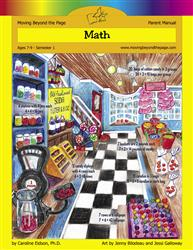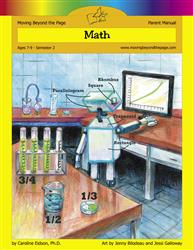# Common Core Alignment

CCSS.Math.Content.3.OA.5 - Apply properties of operations as strategies to multiply and divide.2 Examples: If 6 × 4 = 24 is known, then 4 × 6 = 24 is also known. (Commutative property of multiplication.) 3 × 5 × 2 can be found by 3 × 5 = 15, then 15 × 2 = 30, or by 5 × 2 = 10, then 3 × 10 = 30. (Associative property of multiplication.) Knowing that 8 × 5 = 40 and 8 × 2 = 16, one can find 8 × 7 as 8 × (5 + 2) = (8 × 5) + (8 × 2) = 40 + 16 = 56. (Distributive property.)

## 5: MathUnit 1: Multiplication and Division I
Lesson 10: More Practice With 2, 3, 4, and 5
Lesson 11: Multiples of 10
Lesson 12: Problem Solving With Multiplication
Lesson 14: Multiplication and Division Fact Families
Lesson 15: Unit Test
Lesson 6: The Commutative Property of Multiplication
Lesson 7: Multiples of 2 and 3
Lesson 8: More Practice With 2 and 3
Lesson 9: Multiples of 4 and 5
Final Project: Multiples Mini-PostersUnit 4: Multiplication and Division II
Lesson 1: Mastering More Multiplication Facts
Lesson 12: Unit Test
Lesson 5: Associative Property of Multiplication
Lesson 6: Distributive Property of Multiplication
Lesson 7: Reviewing Properties of Multiplication
Lesson 9: Multiplication and Division Facts Practice
Final Project: Planning a PicnicUnit 9: Skills Review
Lesson 1: Reviewing Multiplication and Division